# Difference between revisions of "Mahler measure"

Given a polynomial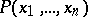with complex coefficients, the logarithmic Mahler measureis defined to be the average over the unit-torus of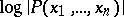, i.e.The Mahler measure is defined by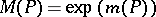, so that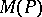is the geometric mean of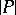over the-torus. Ifand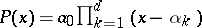, Jensen's formula gives the explicit formulaso that.

The Mahler measure is useful in the study of polynomial inequalities because of the multiplicative property. The important basic inequality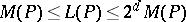[a9] relatesto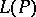, the sum of the absolute values of the coefficients of, wheredenotes the total degree of, i.e. the sum of the degrees in each variable separately. A recent inequality for polynomials of one variable is that, where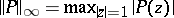,is the sum of the degrees ofand, and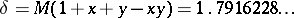is the best possible constant [a2].

Specializing to polynomials with integer coefficients, in case,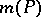is the logarithm of an algebraic integer (cf. Algebraic number). If, there are few explicit formulas known, but those that do exist suggest thathas intimate connections with-theory. For example,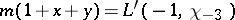, where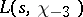is the Dirichlet-function for the odd primitive character of conductor, i.e., and it has been conjectured that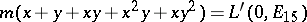, where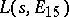is the-function of an elliptic curve of conductor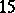. This formula has not been proved but has been verified to overdecimal places [a3], [a4].

The Mahler measureoccurs naturally as the growth rate in many problems, for example as the entropy of certain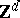-actions [a10]. The set offor which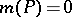is known: in case, a theorem of Kronecker shows that these are products of cyclotomic polynomials and monomials. In case, these are the generalized cyclotomic polynomials [a1]. An important open question, known as Lehmer's problem, is whether there is a constantsuch that if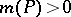, then. This is known to be the case ifis a non-reciprocal polynomial, where a polynomial is reciprocal ifis a monomial. In this case,, where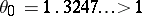is the smallest Pisot number, the real root of[a6], [a1]. A possible value for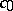is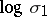, whereis the smallest known Salem number, a number of degree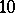known as Lehmer's number.

For, the best result in this direction is that, where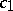is an explicit absolute constant andis the degree of[a5]. A result that applies to polynomials in any number of variables is an explicit constantdepending on the numberof non-zero coefficients ofsuch that[a7], [a1].

A recent development is the elliptic Mahler measure [a8], in which the torus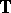is replaced by an elliptic curve. It seems likely that this will have an interpretation as the entropy of a dynamical system but this remains as of yet (1998) a future development.

How to Cite This Entry:
Mahler measure. Encyclopedia of Mathematics. URL: http://encyclopediaofmath.org/index.php?title=Mahler_measure&oldid=24105
This article was adapted from an original article by David Boyd (originator), which appeared in Encyclopedia of Mathematics - ISBN 1402006098. See original article